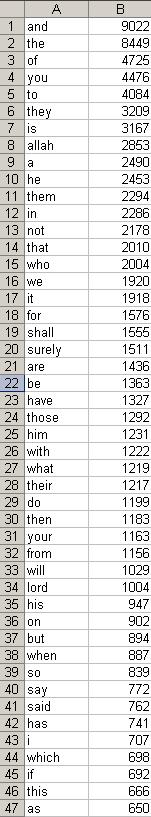# Visualizing Project Euler Problem 58 using Processing/JavaAnother visualization of Euler 58. This time, using Processing with Java.
Previous .NET winforms visualization of Euler 58
https://projecteuler.net/problem=58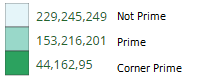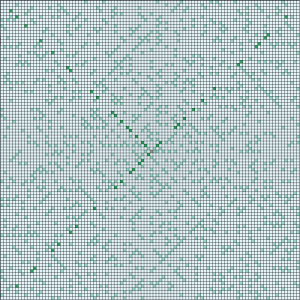```public int i_x = 500; public int i_y = 500; public static int Current_Direction = 0; public int cur_step = 1; public int skip_at = 7; public int skip = 3; public boolean first_turn = false; public int count = 5; public int diag_count = 4; public int prime_count = 2;   void setup() { size(1000, 1000); background(153); }   void draw(){ cur_step += 1; if ( isPrime(cur_step)) { if (cur_step == skip_at) { fill(44,162,95); } else { fill(153,216,201); } } else { fill(229,245,249); }   if (Current_Direction == 0) { rect( i_x + 10, i_y, 10, 10); i_x += 10; } else if (Current_Direction == 3) { rect( i_x, i_y + 10, 10, 10); i_y += 10; } else if (Current_Direction == 2) { rect( i_x - 10, i_y, 10, 10); i_x -= 10; } else if (Current_Direction == 1) { rect( i_x, i_y - 10, 10, 10); i_y -= 10; }   if (cur_step &lt;= 3) Turn(); if (cur_step == 5)//2 Turn(); if (skip_at == cur_step) { skip_at += skip; if (first_turn == false) first_turn = true; else { first_turn = false; skip += 1; } Turn(); } }   protected void Turn() { if (Current_Direction == 3) Current_Direction = 0; else Current_Direction += 1; }   public boolean isPrime(int n) { if (n == 1) return false; if (n == 2) return true; for (int i = 2; i &lt; n; ++i) { if ((n % i) == 0) return false; } return true; }```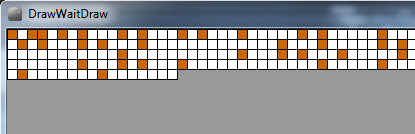A fun little project whilst attempting to learn https://processing.org/

I draw 10 x 10 pixel boxes, 100 per row. If the number is a Prime, I highlight it in orange.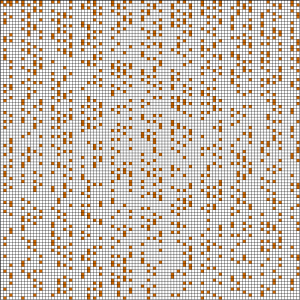Java

```//Number Counter public int i_num = 0; //Draw Coordinates public int i_x = 0; public int i_y = 0;   void setup() { size(1000, 1000); background(153); }   void draw(){   if ( isPrime(i_num)) { fill(204, 102, 0); rect(i_x*10,i_y + 0,10,10); } else { fill(255, 255, 255); rect(i_x*10,i_y + 0,10,10); } i_x +=1; i_num +=1;   //Go to Next Line if ( i_x == 100 ) { i_x = 0; i_y += 10; }   }   public boolean isPrime(int n) { if (n == 1) return false; if (n == 2) return true; for (int i = 2; i &lt; n; ++i) { if ((n % i) == 0) return false; } return true; }```

# Java: LOL!: Gamification of a DataSET :D

Randomized multiple choice quiz of the relationship sets of Fallout 2 Characters and their Locations.

(Character (is from/is related to) Location)
(Concept 1 -> Relationship -> Concept 2)
Example:
( Sulik from Klamath)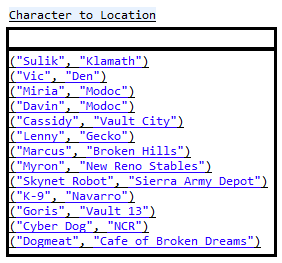Now we want to ask a random question, so we get a random number between 1 and 13 , and pick that row in the data set.

We roll the dice and get “1” so we take the first data row and ask

“Where is Sulik Found?”

This quiz is going to be multiple choice of 4, so we need 3 random incorrect answers, and we need to randomize the correct answer’s location somewhere within the set of incorrect answers.

Make a List of 3 unique/distinct incorrect answers out of the remaining values within the hashtable.  Again, exclude the correct answer  and handle any duplicates.  So in Sulik‘s case, the distinct set of potential incorrect answers looks like this.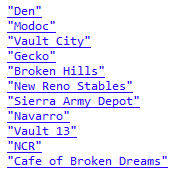Pick another random number and pick 3 distinct values from this list.  Then, append the 3 incorrect values with the correct one.  Pick another random random number 1-4 and display the randomized answers 4 times for “a.)” through “d.)“.

code for now…

```import java.util.Enumeration; import java.util.Hashtable; import java.util.LinkedList; import java.util.ListIterator; import java.util.Random;   public class QuizStructure { public static void main(String[] args) { LinkedList EveryPotentialQuestion_AnswerSet = new LinkedList();   //HOW TO MAKE A Randomized QUIZ off OF a DataSet   //Gamification of a DataSET// LOL :D   System.out.println("indeed."); Hashtable Character_to_Location = new Hashtable();   //"FALLOUT 2 Black Isle Character to Location" Relationship// //( Character - Is_From - Location )// //( Concept - Relationship - Concept ) Character_to_Location.put("Sulik", "Klamath"); Character_to_Location.put("Vic", "Den"); Character_to_Location.put("Miria", "Modoc"); Character_to_Location.put("Davin", "Modoc"); Character_to_Location.put("Cassidy", "Vault City"); Character_to_Location.put("Lenny", "Gecko"); Character_to_Location.put("Marcus", "Broken Hills"); Character_to_Location.put("Myron", "New Reno Stables"); Character_to_Location.put("Skynet Robot", "Sierra Army Depot"); Character_to_Location.put("K-9", "Navarro"); Character_to_Location.put("Goris", "Vault 13"); Character_to_Location.put("Cyber Dog", "NCR"); Character_to_Location.put("Dogmeat", "Cafe of Broken Dreams"); System.out.println(Character_to_Location.toString()); Enumeration keys = Character_to_Location.keys(); while( keys.hasMoreElements() ) { Object key = keys.nextElement(); Object value = Character_to_Location.get(key); System.out.println("Question/Answer Preview: Where is "+key+" from in Fallout 2? Answer : "+value); } Random randomGenerator = new Random(); System.out.println("size: "+Character_to_Location.size()); System.out.println("___________________________________"); //ask Ten randomized questions with 4 randomized answers for( int i = 0 ; i &lt; 10 ; i++) { int randomInt = randomGenerator.nextInt(Character_to_Location.size()); randomInt++; int iStop = 0; Enumeration morekeys = Character_to_Location.keys();   while( morekeys.hasMoreElements() ) { Object key = morekeys.nextElement(); Object value = Character_to_Location.get(key); if ( iStop == randomInt) { //System.out.println("index:" + iStop + " "+randomInt); System.out.println(); System.out.println("Where is "+key+" from?"); //Generate Multiple Choice //we know the correct answer is System.out.println(" Correct Answer : " + value); //now we need to generate other "incorrect" Choices //we need 3, Multiple Choice is Typically 4 questions LinkedList wrongAnswerList = new LinkedList(); //while wrongAnswerList is not unique //we want to remove the possibility of duplicates //we want to not include correct answer boolean is_wronglist_unique = false;   while(!is_wronglist_unique) { int randomWrong = randomGenerator.nextInt(Character_to_Location.size()); randomWrong++; Enumeration wrongkeys = Character_to_Location.keys(); int iStopInner =0; while( wrongkeys.hasMoreElements() ) { Object key2 = wrongkeys.nextElement(); Object value2 = Character_to_Location.get(key2); if ( iStopInner == randomWrong) { if ( !wrongAnswerList.contains(value2)) { if(!value2.equals(value)) wrongAnswerList.add(value2); }   } iStopInner++; } if (wrongAnswerList.size() ==3) {is_wronglist_unique = true;} } System.out.println(" Incorrect Answers : "+wrongAnswerList.toString()); System.out.println(); wrongAnswerList.add(value); System.out.println(); String [] AnswerSet = (String[]) wrongAnswerList.toArray(new String); //get 4 random ints :D //0-3 LinkedList numberset = new LinkedList(); boolean isnumbersetunique = false; int deerpCount = 0; while(!isnumbersetunique) { int randomfinal4 = randomGenerator.nextInt(4); if (!numberset.contains(randomfinal4)) { numberset.add(randomfinal4); deerpCount++; } if(deerpCount == 4) { isnumbersetunique = true; String individualquestion =""; System.out.println("** FINAL QUESTION *** Where is "+key+" from?"); individualquestion += "** FINAL QUESTION *** Where is "+key+" from?"; ListIterator itr = numberset.listIterator(); String[] abcd = {"a.)","b.)","c.)","d.)"}; int ix = 0; while(itr.hasNext()) { int get =(int) itr.next(); System.out.println(" " +abcd[ix]+" "+ AnswerSet[get]); individualquestion += " " +abcd[ix]+" "+ AnswerSet[get]; ix++; }   if ( !EveryPotentialQuestion_AnswerSet.contains(individualquestion)) { EveryPotentialQuestion_AnswerSet.add(individualquestion); //how do I know when this ^ is full ?????? ??????? //What is the most optimal way to answer this ^ without doing it manually? } } } wrongAnswerList.clear(); } iStop++; } } //the more questions you ask the more answers you'll get } }```

Output Example: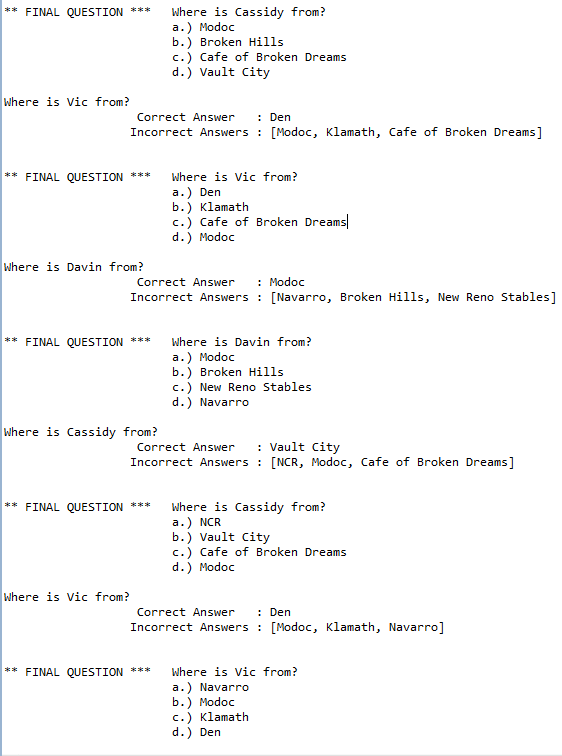What is the total number of distinct Questions and Possible combinations of Answers?  How many unique questions could be asked? Submit your answers below :D

# Java: How to invert a Hashtable?

Say you have a Hashtable with : number for keys, and a comma deliminated list of letters of values.  Now you want to make another hash table which is the opposite.  The keys for the second Hashtable will be a distinct list of all possible letters from the first Hashtable, and the values will be a comma deliminated list of all the numbers associated with a particular letter.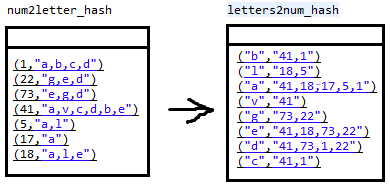```import java.util.Enumeration; import java.util.Hashtable;   public class HashInversion { public static void main(String[] args) {   //hashtable : numbers with lists of letters Hashtable num2letter_hash = new Hashtable();   //hashtable letters with list of numbers Hashtable letters2num_hash = new Hashtable();   num2letter_hash.put(1,"a,b,c,d"); num2letter_hash.put(22,"g,e,d"); num2letter_hash.put(73,"e,g,d"); num2letter_hash.put(41,"a,v,c,d,b,e"); num2letter_hash.put(5,"a,l"); num2letter_hash.put(17,"a"); num2letter_hash.put(18,"a,l,e");   //loop through all the elements in num2letter_hash Enumeration keys = num2letter_hash.keys(); while( keys.hasMoreElements() ) { int key = (int) keys.nextElement(); String content = (String) num2letter_hash.get(key); //break up the content by commas String [] letters = content.split(","); for( int i = 0 ; i &lt; letters.length; i++) { //if this letter hasn't been added to the new hash, // add it, and set its value to the current key //if it already exists in the new table, obtain the current value string // and append a new number/key to it. if( letters2num_hash.containsKey(letters[i].toString()) ) { String previous_num = (String) letters2num_hash.get(letters[i].toString()); letters2num_hash.put(letters[i].toString(), previous_num+","+key); } else { letters2num_hash.put( letters[i].toString(), ""+key); } } } //before (numbers,letter-list) System.out.println(num2letter_hash.toString()); //after (letters,number-list) System.out.println(letters2num_hash.toString()); } }```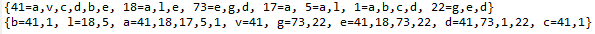# Java: Protege Frames: How to get a distinct list of all possible Slots within a Project.

If for whatever reason you might need this list. All you have to do is loop through the Cls’s, then loop through the Slots of the Cls’s, using the method .getOwnSlots()

More Protege Frames how-to’s here http://mantascode.com/?p=507

```import java.util.ArrayList; import java.util.Collection; import java.util.HashSet; import java.util.Iterator;   import edu.stanford.smi.protege.model.Cls; import edu.stanford.smi.protege.model.KnowledgeBase; import edu.stanford.smi.protege.model.Project;   //This Program will output a complete list of distinct slots within a Protege Frames Project file.   public class jokke { private static final String PROJECT_FILE_NAME = "C:\\MC\\RadLex.pprj"; public static void main(String[] args) { HashSet idsLoaded = new HashSet(); Collection errors = new ArrayList(); Project project = new Project(PROJECT_FILE_NAME, errors); KnowledgeBase kb = project.getKnowledgeBase(); ArrayList distinctSlotList = new ArrayList(); //get Class iterator Iterator radClsIter = kb.getClses().iterator(); //loop through each class while ( radClsIter.hasNext()) { //get Cls object Cls currentClass = (Cls) radClsIter.next(); //String for a comma deliminated list of slots String slotsString = currentClass.getOwnSlots().toString(); String [] clsSlotComponents = slotsString.split(","); //loop through each slot for ( int i = 0 ; i &lt; clsSlotComponents.length; i++ ) { //check to see if particular slot already exists in ArrayList if ( distinctSlotList.contains(clsSlotComponents[i].trim().replaceAll("\\[|\\]", ""))) {} else { distinctSlotList.add(clsSlotComponents[i].trim().replaceAll("\\[|\\]", "")); } } }   Iterator iterator = distinctSlotList.iterator(); while(iterator.hasNext()) { System.out.println(iterator.next()); } } }```

# JAVA: How to programmatically scale down all images in a directory using ImageMagick?

Images are in C:\images
ImageMagick executable is in C:\ImageMagick\convert.exe

Loop through all files in folder and scale them down 25% of their original size.

Use this command to resize the image and append “s_” to the begining of the file name.

Example:
C:\ImageMagick\convert.exe C:\images\1.jpg -resize 25% C:\images\s_1.jpg

```import java.io.File; import java.io.IOException;   public class ImgManipulator { public static void main(String[] args) { String path = "c:\\images\\"; String files; File folder = new File(path); File[] listOfFiles = folder.listFiles(); for (int i = 0; i &lt; listOfFiles.length; i++) { if (listOfFiles[i].isFile()) { files = listOfFiles[i].getName(); System.out.println("Scaling"+files); try { Process p = Runtime.getRuntime().exec( "C:\\ImageMagick\\convert.exe C:\\images\\"+files+" -resize 25% C:\\images\\s_"+files); } catch (IOException e) { e.printStackTrace(); } } } }   }```

# JAVA: How to programmatically manipulate a Protégé-Frames lexicon / ontology / dictionary using Protege API and Java.

This tutorial will give a brief overview of how to modify a Protégé-Frames dictionary using Java.

protege.stanford.edu/

Reference the API:

protege.stanford.edu/protege/3.4/docs/api/core/

The Following examples will require these imports:

```import edu.stanford.smi.protege.model.Cls; import edu.stanford.smi.protege.model.Instance; import edu.stanford.smi.protege.model.KnowledgeBase; import edu.stanford.smi.protege.model.Project; import edu.stanford.smi.protege.model.Slot;```

Assuming you’ve successfully associated the appropriate Protege libraries with your Eclipse, lets get started with some simple HOW-TOs in programmatical Protege ontology manipulation;

For this tutorial I will be using the RadLex Lexicon/Dictionary.

The first thing you will need is the actual project files.
These files are created when you save a Lexicon/Dictionary in Frames format using Protege.

Create a pointer to them in your java code. I have mine in in C:\smelo\RadLex.pprj
You only have to point to the *.pprj file.

```//Get the project file of the lexicon you want to manipulate private static final String PROJECT_FILE_Pointer = "C:\\smelo\\RadLex.pprj";```

Next, you will need to get the KnowledgeBase object.

```//errors object is required in getting the project Collection errors = new ArrayList(); //Get Project project Project project = new Project(PROJECT_FILE_Pointer, errors); //Get Knowledgebase kb KnowledgeBase kb = project.getKnowledgeBase();```

In Protege Frames, each Term/Concept/Lexicon Entity/Thingy is considered a “class”(Cls). The entire RadLex dictionary is nothing more then a bunch of terms(classes) with attributes(slots), and the relationships amongst them.

So lets iterate through all the classes in the KnowledgeBase.

To do this we need to get a class iterator:

```//RadLex Class iterator Iterator radClsIter = kb.getClses().iterator();```

Loop through every class

In RadLex, the “name” of every class is its Radlex Id number, or “RID###..”
such as “RID1099” “RID35707” “RID3874” ….

Every RadLex class contains a number of “slots” or attributes that you can populate:

Using the method .getOwnSlots() on a Cls Object will show you a list of all the slots and their names

These are all different attributes(slots) of a single concept(Cls) in RadLex:

Slot(Related_Condition), Slot(Anatomical_Site), Slot(Related_modality), Slot(ACR_ID), Slot(UMLS_Term), Slot(Is_A), Slot(UMLS_ID), Slot(Misspelling of term), Slot(Has_Subtype), Slot(Non-English_name), Slot(Member_Of), Slot(Preferred_name), Slot(Source), Slot(Non-Sanctioned Synonym), Slot(Synonym), Slot(Version_Number), Slot(Term_Status), Slot(SNOMED_ID), Slot(Acronym), Slot(SNOMED_Term), Slot(ACR_Term), Slot(Comment), Slot(Image_URL), Slot(Definition), Slot(:ROLE), Slot(:DOCUMENTATION), Slot(:SLOT-CONSTRAINTS), Slot(:DIRECT-INSTANCES), Slot(:DIRECT-SUPERCLASSES), Slot(:DIRECT-SUBCLASSES), Slot(:DIRECT-TEMPLATE-SLOTS), Slot(:NAME), Slot(:DIRECT-TYPE)

Some slot types are simple strings, while others are more complex Instances.

```while( radClsIter.hasNext() ) { Cls currentClass = radClsIter.next(); }```

So now that we have a brief scattered overview of the RadLex Lexicon/Dictionary,
and we know how to iterate through each Concept/Term/Class within it. Lets do some practical HOW-TOs.

HOW TO get the value of “Preferred_name” slot from a class object(currentClass)?

One way of retrieving of the content of a slot of a class would be by the slot’s name.
So all you have to do is, get another slot iterator this time for the class(term/concept), and compare its name with the one you want.
Then, iterate through the content of the slot and display its value.(You might not have to do this last step; it depends of the type of slot)

In RadLex the Preferred_name slot of a class is populated with an Instance type.
So we have to get the instance of a slot then get the name of the Instance.

```String slotName = "Preferred_name";   Iterator slotIter = currentClass.getOwnSlots().iterator();   //Iterate through the slots while( slotIter.hasNext() ) { Slot currentSlot = slotIter.next();   //Compare the slot name with your slotName String if( slotName.equals( currentSlot.getName() ) ) { Object slotContentObject = null;   //Create another iterator for the content of the slot Iterator iterSlotContent = currentClass.getOwnSlotValues(currentSlot).iterator();   if( iterSlotContent.hasNext() ) { slotContentObject = iterSlotContent.next();   //if the content of a slot is of type Instance if(slotContentObject instanceof Instance) { //cast to Instance Instance instSlotValue = (Instance) slotContentObject;   //use the method getBrowserText() on the Instance to get its String content System.out.println(instSlotValue.getBrowserText()); } } } }```

HOW TO change the Definition slot of a Class/Concept/Term by specific “name” (RID) and save to project file?

Say we want to change the content of the “Definition” slot in Cls “RID35712”:

```String slotName = "Definition";   //get the Cls object of the Term/Concept/Class Cls myConcept = kb.getCls( "RID35712" );   Iterator myConceptClsIter = myConcept.getOwnSlots().iterator(); while( myConceptClsIter.hasNext() ) { Slot currentSlot = myConceptClsIter.next(); if(slotName.equals(currentSlot.getName()) ) { //set new Definition myConcept.setOwnSlotValue(currentSlot, "This is my new Definition for Term RID35712"); } }   //save changes project.save(errors);```

HOW TO display ALL synonyms of the entire project?

What if we want to list the names of ALL synonyms throughout the entire dictionary

```Iterator radClsIter = kb.getClses().iterator();   while( radClsIter.hasNext() ){ Cls currentClass = radClsIter.next(); String prefName = findPreferredName(currentClass);   String slotName = "Synonym"; Iterator slotIter = currentClass.getOwnSlots().iterator();   while( slotIter.hasNext() ) { Slot currentSlot = slotIter.next(); if(slotName.equals(currentSlot.getName()) ) { Object slotContentObject = null; Iterator iterSlotContent = currentClass.getOwnSlotValues(currentSlot).iterator(); if( iterSlotContent.hasNext() ) { slotContentObject = iterSlotContent.next(); if(slotContentObject instanceof Instance) { Instance instSlotValue = (Instance) slotContentObject; System.out.println(instSlotValue.getBrowserText()); } } } } }```

HOW TO create a new Class/Concept/Term and Add it as a child of RID35712?

We will be assigning the “name” as new unique “RID12345” and adding it as child of the term “RID35712”

```Collection myCol = kb.getClsNameMatches("RID35712",1); kb.createCls("RID12345", myCol);```

That covers a few basics, if you would like an example of something I havent covered, feel free to leave a comment.

GL & HF :D

# JAVA: How to parse every word in a book and output the frequency of repeating words: The Holy Koran

The book being parsed in this example is the Holy Koran.  (English Translation) Koran.txt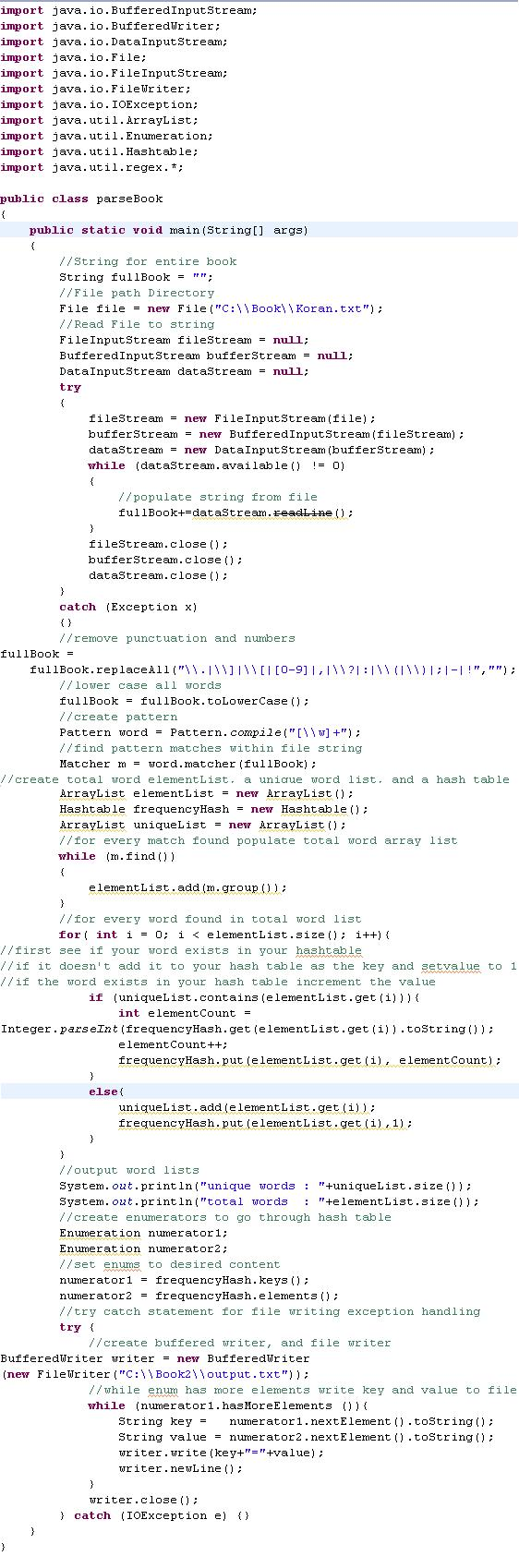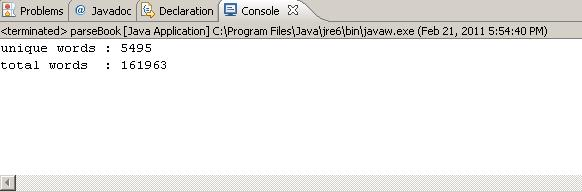Column A is the word, Column B is the frequency